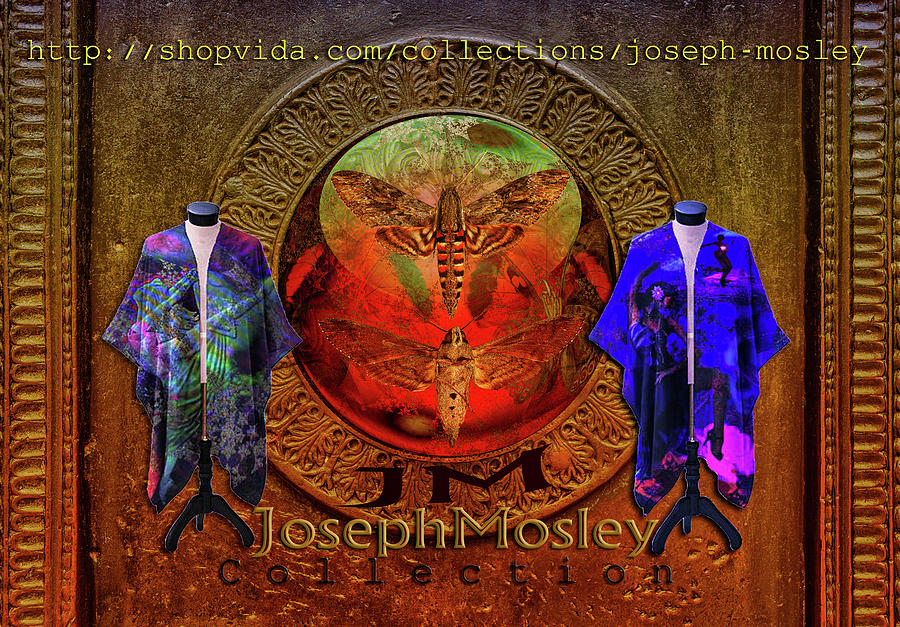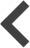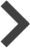# Joseph Mosley CollectionPewaukee, WI - United States

FONT ART LF GROUP FEATURE...The Artist Who Was Formerly Known As Six thank"U";-)

Tallahassee, FL - United States

Joseph, thank you for posting this featured book cover to FONT ART. LF

Pewaukee, WI - United States

R e b e c c a M a l o T h a n k"U"C o n t e m p o r a r y G r o u p F e a t u r e...

Pewaukee, WI - United States

Thanks! Mr Nader Rangidan;-)

Pewaukee, WI - United States

Thank"U";-)Ian Gledhill;-))))

Pewaukee, WI - United States

Ian Gledhill " A w e s o m e A s i a G r o u p f e a t u r e...Thank"U" ;-)

Pewaukee, WI - United States

Roxane Gabriel" S p i r i t u a l S u r r e a l i s m G r o u p F e a t u r e...Thank"U" R o x a n e ;-)

Pewaukee, WI - United States

Nader Randigan" F i n e A r t s P r o f e s s i o n a l s G r o u p f e a t u r e...Thank"U" N. R.

Pewaukee, WI - United States

John M. Bailey "I m a g e s t h a t E x c i t e Y o u G r o u p F e a t u r e...t h n a k"U" J o h n ;-)

Pewaukee, WI - United States

D e n i s e D a v i s " A l l A s p e c t s O F A b s t r a c t A r t G r o u p F e a t u r e ...T h a n k "U" D e n i s e ;-) D a v i s

Pewaukee, WI - United States

Romuald Henry Wasielewski "A r t s F a n t a s t i c W o r l d G r o u p F e a t u r e ...T h a n k"U" R.H.W. ;-)

Pewaukee, WI - United States

T a n y a L o z a n o -T u l " A b s t r a c t M o o d s G r o u p f e a t u r e...T h a n k y o u! M s T a n y a L o z a n o -T u l ;-)

Pewaukee, WI - United States

J e n n P o i n t e r " F i g u r a t i v e A b s t r a c t i o n G r o u p F e a t u r e...T h a n k y o u! M s. J e n n P o i n t e r ;-)

Pewaukee, WI - United States

Thank"U";-)VIVA A n d e r s o n very a p p r e c i a t e d...

Mosman-Sydney, NS - Australia

Beautifully conjured/presented, Josef.......my compliments.........much symbolism here to relate to......thank you........fv..........VIVA